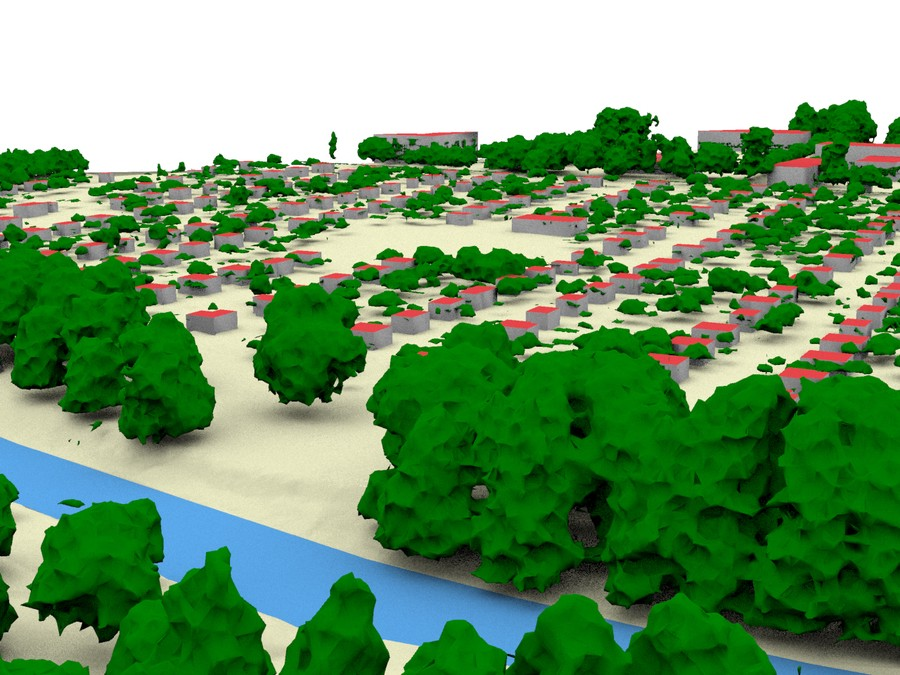# 3D modeling of vegetation

Segmentation and 3D reconstruction of vegetation from LiDAR point cloudsThis research started with need for an accurate 3D modeling of vegetation for solar irradiation. A preliminary study showed that the effect of vegetation on façade solar irradiation is significant.

Subsequent studies refined the 3D modeling with alpha shapes reconstruction, also implemented using the CGAL Library. Here’s the Windows executable that can be used with a `*.xyz` input file in the command line to produce a 3D mesh of trees:

``alphashape.exe alpha infile.xyz ``

where `alpha` is the alpha parameter (e.g. 0.75) and `infile.xyz` is the input file.

This Github repository contains some utilities (MATLAB and C++) for the 3D reconstruction of vegetation from point clouds.